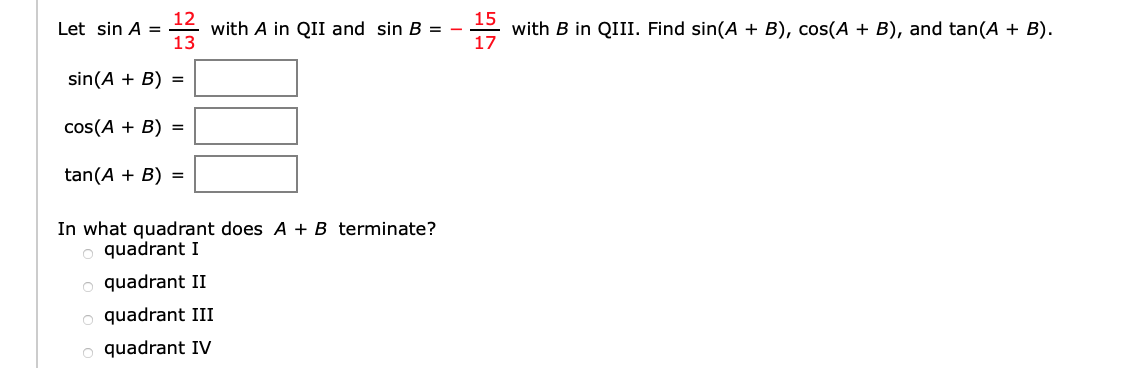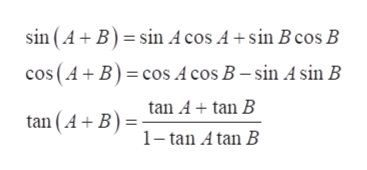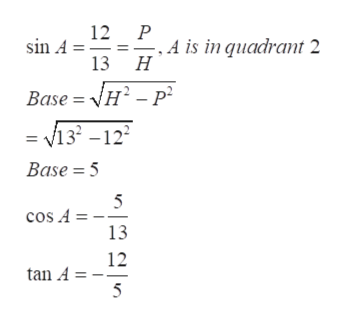# 12with A in QII and sin B = -1315with B in QIII. Find sin(A + B), cos(A + B), and tan(A + B)17Let sin A =sin(AB)cos(AB)tan(AB)In what quadrant does A + B terminate?quadrant Iquadrant IIquadrant IIIquadrant IV

Question
3 viewshelp_outlineImage Transcriptionclose12 with A in QII and sin B = - 13 15 with B in QIII. Find sin(A + B), cos(A + B), and tan(A + B) 17 Let sin A = sin(AB) cos(AB) tan(AB) In what quadrant does A + B terminate? quadrant I quadrant II quadrant III quadrant IV fullscreen
check_circle

Step 1

Refer to the question, we have to find the value of sin(A+B) , cos(A+B) and tan(A+B) when provided with sin A=12/13 with A in Q2 and Sin B=-15/17 with B in Q3.

Step 2

Now use the formula for the identity  sin (A+B), cos (A+B) and tan(A+B).help_outlineImage TranscriptioncloseB sin Acos A +sin Bcos B sin (A cos (A B) cos A cos B - sin A sin B tan Atan B tan (A B) 1-tan A tan B fullscreen
Step 3

Now as we know that in first quadrant all the trigonometric ratios are positive , in second only sine and cosec is positive rest are negative, in thir...help_outlineImage Transcriptionclose12 P sin A ., A is in quacrant 2 13 H Base = H2-P = v133 -12 Base 5 5 cos A = 13 12 tan A 5 fullscreen

### Want to see the full answer?

See Solution

#### Want to see this answer and more?

Solutions are written by subject experts who are available 24/7. Questions are typically answered within 1 hour.*

See Solution
*Response times may vary by subject and question.
Tagged in

### Trigonometric Ratios